# Accounting Ratios (Part-4) Notes | Study TS Grewal Solutions - Class 12 Accountancy - Commerce

## Commerce: Accounting Ratios (Part-4) Notes | Study TS Grewal Solutions - Class 12 Accountancy - Commerce

The document Accounting Ratios (Part-4) Notes | Study TS Grewal Solutions - Class 12 Accountancy - Commerce is a part of the Commerce Course TS Grewal Solutions - Class 12 Accountancy.
All you need of Commerce at this link: Commerce

Question:76

From the following Information, calculate Inventory Turnover Ratio:

Credit Revenue from Operations  3,00,000; Cash Revenue from Operations  1,00,000, Gross Profit 25% of Cost, Closing Inventory was 3 times the Opening Inventory. Opening Inventory was 10% of Cost of Revenue from Operations.

Solution:

Average Inventory = (30,000+90,000)/2 = Rs 60,000

Opening Inventory = 3,00,000 × 10% = Rs 30,000

Closing Inventory = 30,000 × 3 = Rs 90,000

Cost of Revenue from Operations = Revenue from Operations - Gross Profit

= 4,00,000-1,00,000 = Rs 3,00,000

Inventory Turnover Ratio = Cost of Revenue from Operations/Average Inventory

= 3,00,000/60,000 = 5 times

Question:77

Calculate Inventory Turnover Ratio in each of the following alternative cases:

Case 1: Cash Sales 25% of Credit Sales; Credit Sales 3,00,000; Gross Profit 20% on Revenue from Operations, i.e., Net Sales; Closing Inventory 1,60,000; Opening Inventory 40,000.

Case 2: Cash Sales 20% of Total Sales; Credit Sales 4,50,000; Gross Profit 25% on Cost; Opening Inventory 37,500; Closing Inventory 1,12,500.

Solution:

Case 1

Credit Sales = 3,00,000

Cash sales = 25% of Credit Sales

Cash sales  = 3,00,000 x 25/100 = 75,000

Total Sales = Cash Sales + Credit Sales = 3,00,000 + 75,000 = 3,75,000

Gross Profit = 20% on Sales

Gross Profit = 3,75,000 x 20/100 = 75,000

Cost of Goods Sold = Total Sales − Gross Profit = 3,75,000 − 75,000 = 3,00,000

Average Stock = (Opening Stock + Closing Stock)/2

= (40,000 + 1,60,000)/2 = Rs 1,00,000

Inventory Turnover ratio = Costs of Goods sold/Average Inventory = 3,00,000/1,00,000 = 3 times
Case 2

Cash sales = Total sales*20/100

Cash sales = x*20/100

Total Sales = Cash Sales + Credit Sales

x = 20x/100 + 4,50,000

x - 20x/100 = 4,50,000

x = 5,62,000

Let cost of good sold = a

Gross profit = a*25/100 = 25a/100

Gross Profit = Sales − Cost of Goods Sold

25a/100 = 5,62,000 - a

a = 4,50,000

Cost of good solds = a = 4,50,000

Average Stock = (Opening Stock + Closing Stock)/2

= (37,500 + 1,12,500)/2 = Rs 75,000

Stock Turnover Ratio = Costs of Goods sold/Average Stock = 4,50,000/75,000 = 6 times

Question:78

From the following Statement of Profit and Loss for the year ended 31st March, 2019 of Rex Ltd., calculate Inventory Turnover Ratio: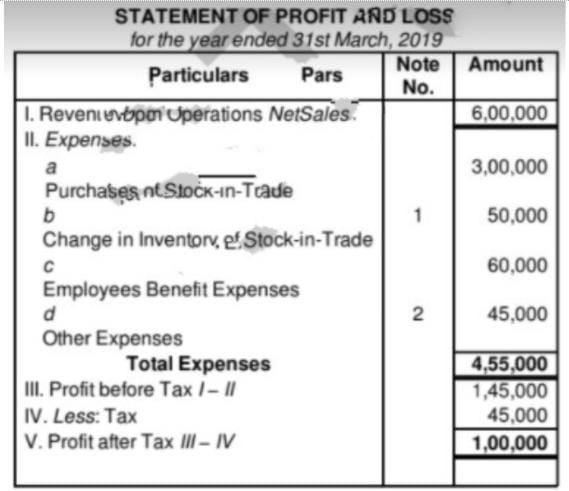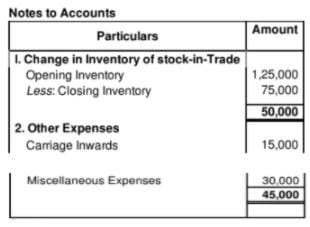Solution:
Cost of Goods Sold = Opening Inventory Purchases + Direct Expenses – Closing Inventory  = Rs 1,25,000 + Rs 3,00,000 + Rs 15,000 – Rs 75,000 = Rs 3,65,000
Average Stock = (Opening Stock + Closing Stock)/2

= (1,25,000 + 75,000)/2 = Rs 1,00,000

Inventory Turnover ratio = Costs of Goods sold/Average Inventory = 3,65,000/1,00,000 = 3.65 times

Question:79

Credit Revenue from Operations, i.e., Net Credit Sales for the

year  - 1,20,000

Debtors - 12,000

Bills Receivable - 8,000

Solution: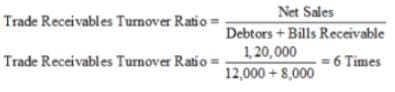Question:80

Calculate Trade Receivables Turnover Ratio from the following information: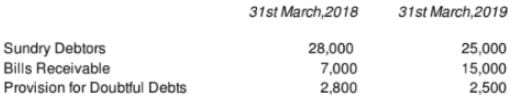Total Sales  1,00,000; Sales Return  1,500; Cash Sales  23,500.

Solution:

Net Credit Sales = Total Sales − Sales Return − Cash Sales

= 1,00,000 − 1,500 − 23,500 = 75,000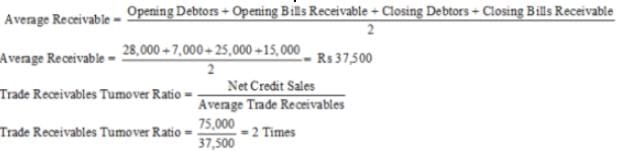Question:81

Closing Trade Receivables  1,00,000; Cash Sales being 25% of Credit Sales; Excess of Closing Trade Receivables over Opening Trade Receivables  40,000; Revenue from Operations, i.e., Net Sales  6,00,000. Calculate Trade Receivables Turnover Ratio.

Solution:

Let Credit Sales be = x

Cash sales  = x * 25/100 = 25x/100

Total Sales = Cash Sales + Credit Sales
6,00,000 = 25x/100 + x
x = 4,80,000
Credit Sales = 4,80,000

1,00,000 = Opening Trade Receivables + 40,000

Opening Trade Receivables = Rs 60,000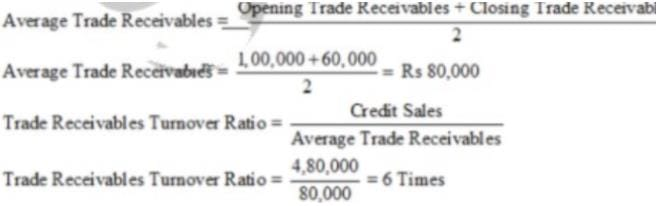Question:82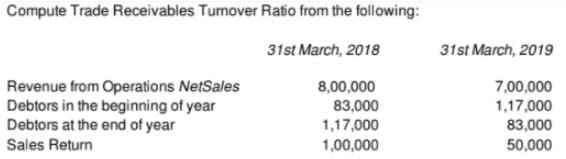Solution: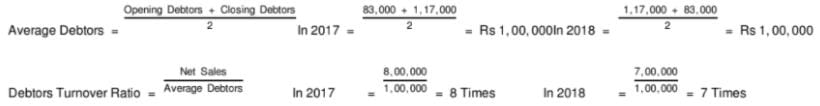Question:83
1,75,000 is the Credit Revenue from Operations, i.e., Net Credit Sales of an enterprise. If Trade Receivables Turnover Ratio is 8 times, calculate Trade Receivables in the Beginning and at the end of the year. Trade Receivables at the end is  7,000 more than that in the beginning.
Solution:
Total Receivables turnover ratio = Net Credit Sales/Average trade receivables
Average trade receivables = Rs. 21,875
Let Opening Trade Receivables = x
Close Trade Receivables = x + 7,000
21,875 = (x + x + 7,000)/2
x = Rs 13,875
∴ Opening Trade Receivables = x = 18,375

Closing Trade Receivables = x +7,000 = 25,375

Question:84

From the following particulars, determine Trade Receivables Turnover Ratio:
Revenue from Operations Net Sales - 10,00,000

Credit Revenue from Operations Credit Sales - 8,00,000

Solution:
Credit Sales = 8,00,000

Average Debtors = 1,00,000
= 8,00,000 /1,00,000 = 8 times

Question:85

Closing Trade Receivables  1,20,000, Revenue from Operations  14,40,000. Provision for Doubtful Debts  20,000. Calculate Trade Receivables Turnover Ratio.
Solution:

Revenue from Operations =  14,40,000

Since, opening trade receivables have not been given we assume closing trade receivables to be our average trade receivables. Also, the revenue from operations will be assumed to be revenue from net credit sales.

Trade Receivables Turnover Ratio = Credit Revenue from Operations/Average Credit receivables

= 14,40,000/1,20,000 = 12 times

Therefore, this higher ratio indicates the rate at which the firm is able to collect its debt efficiently.

Question:86

Closing Trade Receivables  4,00,000; Cash Sales being 25% of Credit Sales; Excess of Closing Trade Receivables over Opening Trade Receivables  2,00,000; Revenue from Operations, i.e., Revenue from Operations, i.e., Net Sales  15,00,000. Calculate Trade Receivables Turnover Ratio

[Hint: 1. Net Credit Sales = Total Sales − Cash Sales

Solution:

Let Credit Sales be = x
Cash sales = 25% of credit sales
Cash sales = 25x/100
Total Sales = Cash Sales + Credit Sales
15,00,000 = 25x/100 + x
x = 12,00,000

= 4,00,000 − 2,00,000 = 2,00,000
= (2,00,000 + 4,00,000)/2 = Rs. 3,00,000
= 12,00,000/3,00,000 = 4 times

Question:87
A firm normally has trade Receivables equal to two months' credit Sales. During the coming year it expects Credit Sales of  7,20,000 spread evenly over the year 12months . What is the estimated amount of Trade Receivables at the end of the year?
Solution:
Debt Collection Period = 12 Months/Debtors’ Turnover ratio
2 = 12/Debtors’ Turnover ratio
Debtors’ Turnover ratio = 6 times
Debtors Turnover Ratio  = Credit sales/Debtors at the end
6 = 7,20,000/Debtors at the end
Debtors at the end = Rs. 1,20,000

Question:88

A limited company made Credit Sales of  4,00,000 during the financial period. If the collection period is 36 days and year is assumed to be 360 days, calculate:

Iii. Trade Receivables at the end when Trade Receivables at the end are more than that in the beginning by  6,000.

Solution:

Total Sales = Cost of Goods Sold + Gross Profit

= 3,50,000 + 1,50,000 = 5,00,000

Credit Sales = Total Sales − Cash Sales

= 5,00,000 − 2,00,000 = 3,00,000
Average Trade Receivables = Rs. 1,00,000
Case 1:
Let Opening Trade Receivables = x

Closing Trade Receivables = x + 1,00,000
1,00,000 = (x + x + 1,00,000)/2
x = 50,000
∴ Opening Trade Receivables = x = 50,000

Closing Trade Receivables = 3x = 1,50,000
Case 2:
Let Opening Trade Receivables = x

1,00,000 = (x + 3x)/2
x = 50,000
∴ Opening Trade Receivables = x = 50,000

Closing Trade Receivables = 3x = 1,50,000
Case 3:
Let Opening Trade Receivables = x

Closing Trade Receivables = x + 3x

1,00,000 = (x + 4x)/2
x = 40,000
∴ Opening Trade Receivables = x = 40,000

Closing Trade Receivables = 4x = 1,60,000

Question:89

Cash Revenue from Operations Cash Sales

2,00,000, Cost of Revenue from Operations or Cost of Goods Solds  3,50,000; Gross Profit  1,50,000; Trade Receivables Turnover Ratio 3 Times. Calculate Opening and Closing Trade Receivables in each of the following alternative cases;

Case 1 : If Closing Trade Receivables were  1,00,000 in excess of Opening Trade Receivables.
Case 2 : If trade Receivables at the end were 3 times than in the beginning.
Case 3 ; If Trade Receivables at the end were 3 times more than that of in the beginning.
Solution:
(i) Debt collection Period = 360/Trade Receivables Turnover Ratio
36 = 360/Trade Receivables Turnover Ratio
Trade Receivables Turnover Ratio = 10 times
(iii) Let the opening Trade receivables be x
Therefore, closing trade receivables = x + 6,000
40,000 = (x + x + 6000)/2
x = 37,000
∴ Opening Trade Receivables = x = 37,000

Closing Trade Receivables = x + 6000 = 43,000

Question:90

From the following information, calculate Opening and Closing Trade Receivables, if Trade Receivables Turnover Ratio is 3 Times:
i. Cash Revenue from Operations is 1/3rd of Credit Revenue from Operations.

Ii. Cost of Revenue from Operations is 3,00,000.

Iii. Gross Profit is 25% of the Revenue from Operations.

Iv. Trade Receivables at the end are 3 Times more than that of in the beginning.

Solution:
Average Trade Receivables = Rs. 1,00,000
1,00,000 = (x + 4x)/2
x = 40,000
∴ Opening Trade Receivables = x = 40,000

Closing Trade Receivables = 4x = 1,60,000
Revenue from operations = 3,00,000 + (25/75)*3,00,000  = Rs 4,00,000
Credit Revenue from Operations = Total Revenue from Operations − Cash Revenue from Operations
x = 4,00,000 - (1/3)x
Credit Revenue from Operations = Rs 3,00,000

Question:91

Calculate Trade Receivables Turnover Ratio in each of the following alternative cases:

Case 1: Net Credit Sales 4,00,000; Average Trade Receivables 1,00,000.

Case 2: Revenue from Operations NetSales 30,00,000; Cash Revenue from Operations, i.e., Cash Sales 6,00,000; Opening Trade Receivables 2,00,000; Closing Trade Receivables 6,00,000.
Case 3: Cost of Revenue from Operations or Cost of Goods Sold 3,00,000; Gross Profit on Cost 25%; Cash Sales 20% of Total Sales; Opening Trade Receivables 50,000; Closing Trade Receivables 1,00,000.
Case 4: Cost of Revenue from Operations or Cost of Goods Sold 4,50,000; Gross Profit on Sales 20%; Cash Sales 25% of Net Credit Sales, Opening Trade Receivables 90,000; Closing Trade Receivables 60,000.

Solution:
Case 1:
= 4,00,000/1,00,000 = 4 times
Case 2:
Net credit sales = Total sales - Cash Sales
= 30,00,000 - 6,00,000 = 24,00,000
= (2,00,000 + 6,00,000)/2 = 4,00,000
= 24,00,000/4,00,000 = 6 times
Case 3:
Cost of Goods Sold = 3,00,000

Gross Profit = 25% on Cost
Gross profit = 25/100 * 3,00,000 = 75,000
Total Sales = Cost of Goods Sold + Gross Profit

= 3,00,000 + 75,000 = 3,75,000
Cash Sales = 20% of Total Sales
Cash sales =  3,75,000*20/100 = 75,000
Credit Sales = Total Sales − Cash Sales

= 3,75,000 − 75,000 = 3,00,000

= (50,000 + 1,00,000)/2 = 75,000

= 3,00,000/75,000 = 4 times
Case 4:
Let Sales be = x
Gross profit = x*20/100 = 20x/100
Sales = Cost of Goods Sold + Gross profit
x = 4,50,000 + 20x/100
Sales = x = Rs 5,62,000
Let credit sales be = a
Cash sales = a*25/100 = 25a/100
Sales = Cash sales + Credit sales
5,62,000 = 25a/100 + a
a = 4,50,000
Credit sales = a = 4,50,000

= (90,000 + 60,000)/2 = 75,000

= 4,50,000/75,000 = 6 times

Question:92

From the information given below, calculate Trade Receivables Turnover Ratio:

Credit Revenue from Operations, i.e., Credit Sales 8,00,000; Opening Trade Receivables 1,20,000; and Closing Trade Receivables 2,00,000.

State giving reason, which of the following would increase, decrease or not change Trade Receivables Turnover Ratio:

i. Collection from Trade Receivables 40,000.

ii. Credit Revenue from Operations, i.e., Credit Sales 80,000.

iii. Sales Return 20,000.

iv. Credit Purchase 1,60,000.
Solution: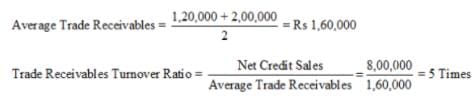i. Collection from Trade Receivables Rs 40,000- Increase

Reason: Collection from Trade Receivables will result in decrease in the amount of closing Trade Receivables which will reduce the amount of average Trade Receivables.

Closing Trade Receivables = 2,00,000 − 40,000 = Rs 1,60,000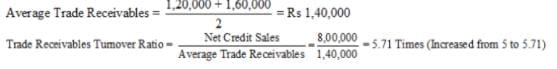ii. Credit Revenue from Operations, i.e. Sales Rs 80,000- Decrease

Reason: This transaction will result in increase in both credit sales as well as closing Trade Receivables. Increase in closing Trade Receivables, in turn, will lead to an increase in the average Trade Receivables.

Credit Sales = 8,00,000 + 80,000 = Rs 8,80,000

Closing Trade Receivables = 2,00,000 + 80,000 = Rs 2,80,000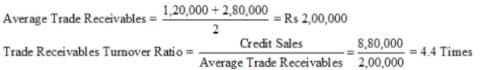iii. Sales Return Rs 20,000- Increase

Reason: This transaction will result in decrease in both sales and average Trade Receivables. Credit Sales = 8,00,000 − 20,000= Rs 7,80,000

Closing Trade Receivables = 2,00,000 − 20,000 = Rs 1,80,000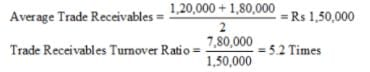iv. Credit Purchase Rs 1,60,000- No Change

Reason: Credit Purchase does not affect the Debtors Turnover Ratio.

Question:93
Calculate Trade Payables Turnover Ratio and Average Debt payment Period from the following information: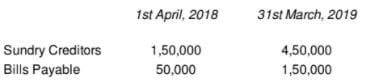Total Purchases  21,00,000; Purchases Return  1,00,000; Cash Purchases  4,00,000.
Solution: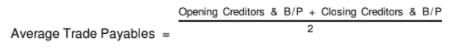= (1,50,000 + 50,000 + 4,50,000 + 1,50,000)/2
= Rs 4,00,000
Net Credit Purchases = Total Purchase - Purchase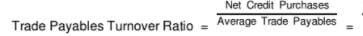16,00,000/4,00,000 = 4 times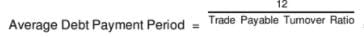= 12/4 = 3 months

Question:94

Calculate Trade payables Turnover Ratio from the following information:

Opening Creditors  1,25,000; Opening Bills Payable  10,000; Closing Creditors  90,000; Closing bills Payable  5,000; Purchases  9,50,000; Cash Purchases  1,00,000; Purchases Return  45,000.
Solution:

Net Credit Purchases = Purchases – Cash Purchases – Purchase Return

= Rs 9,50,000 – Rs 1,00,000 – Rs 45,000 = Rs 8,05,000
Average trade payables = (1,25,000 + 10,000 + 90,000 + 5,000)/2 = Rs 1,15,000
Trade payables Turnover Ratio = 8,05,000/1,15,000 = 7 times

Question:95

Calculate Trade Payables Turnover Ratio for the year 2018-19 in each of the alternative cases:

Case 1 : Closing Trade Payables  45,000; Net Purchases  3,60,000; Purchases Return  60,000; Cash Purchases  90,000.

Case 2 : Opening Trade Payables  15,000; Closing Trade Payables  45,000; Net Purchases  3,60,000.

Case 3 : Closing Trade Payables  45,000; Net Purchases  3,60,000.

Case 4 : Closing Trade Payables

including 25, 000duetoasupplierofmachinery  55,000; Net Credit Purchases  3,60,000.

Solution:
Case 1:
Net Credit Purchases = Net Purchases − Cash Purchases

= 3,60,000 − 90,000 = 2,70,000
Trade Payables Turnover ratio = Net CreditPurchases/Closing Trade Payables = 2,70,000/45,000 = 6 times
Case 2
Net Purchases = 3,60,000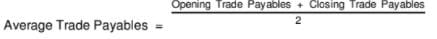= 3,60,000/30,000 = 12 times
Case 3
Trade Payables Turnover Ratio = Net Credit Purchases/Closing Trade Payables = 3,60,000/45,000 = 8 times

Case 4
Net Credit Payables for Goods = Trade Payables − Creditors for Machinery = 55,000 − 25,000 = 30,000
Trade Payables Turnover Ratio = Net Credit Purchases/Closing Trade Payables = 3,60,000/30,000 = 12 times

Question:96
From the following information, calculate Working Capital Turnover Ratio: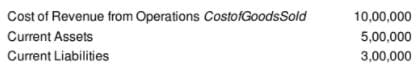Solution:

Working Capital = Current Assets – Current Liabilities

= 5,00,000 – 3,00,000 = 2,00,000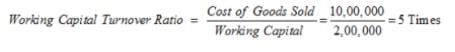Question:97

Revenue from Operations: Cash Sales  5,00,000; Credit Sales  6,00,000; Sales Return  1,00,000. Current Assets  3,00,000; Current Liabilities  1,00,000. Calculate Working Capital Turnover Ratio.
Solution:

Net Sales = Cash Sales + Credit Sales − Sales Returns

= 5,00,000 + 6,00,000 − 1,00,000 = 10,00,000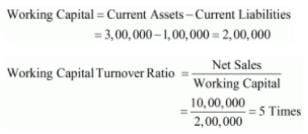Question:98
Equity Share Capital  15,00,000; Gross Profit on Revenue from Operations, i.e., Net Sales 33 1/3%
Cost Revenue from Operations or Cost of Goods Sold  20,00,000; Current Assets  10,00,000; Current Liabilities  2,50,000. Calculate Working Capital Turnover Ratio.
Solution:

Net Sales = Cost of Goods sold + Gross Profit
Net sales = 20,00,000 + ⅓ of net sales

Let Net Sales = x

x = 20,00,000 + x/3
Net sales (x) = 60,00,000/2 = Rs 30,00,000

Working Capital = Current Assets - Current Liabilities

= 10,00,000 - 2,50,000 = 7,50,000
Working capital Turnover ratio = Net Sales/Working Capital

= 30,00,000/7,50,000 = 4 times

Question:99

Gross Profit at 25% on cost; Gross profit  5,00,000; Equity Share Capital  10,00,000; Reserves and Surplus 2,00,000; Long-term Loan 3,00,000; Fixed Assets Net  10,00,000. Calculate Working Capital Turnover Ratio

Solution:

Gross Profit = 25% on Cost

Let cost be = Rs x

Gross profit = 25x/100

5,00,000 = 25x/100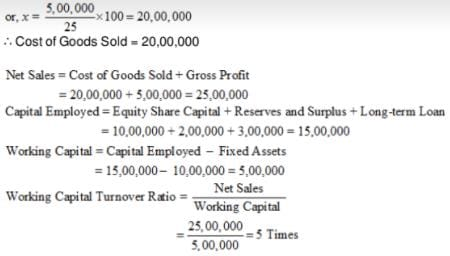Question:100

Capital Employed  12,00,000; Net Fixed Assets 8,00,000; Cost of Goods Sold or Cost of Revenue from Operations  40,00,000; Gross Profit is 20% on Cost. Calculate Working Capital Turnover Ratio.

Solution:

Cost of Goods Sold = 40,00,000

Gross Profit = 20% of Cost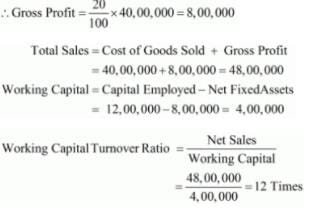The document Accounting Ratios (Part-4) Notes | Study TS Grewal Solutions - Class 12 Accountancy - Commerce is a part of the Commerce Course TS Grewal Solutions - Class 12 Accountancy.
All you need of Commerce at this link: CommerceUse Code STAYHOME200 and get INR 200 additional OFF

## TS Grewal Solutions - Class 12 Accountancy

52 docs

Track your progress, build streaks, highlight & save important lessons and more!

,

,

,

,

,

,

,

,

,

,

,

,

,

,

,

,

,

,

,

,

,

;